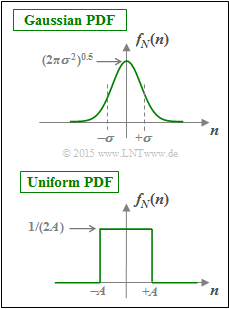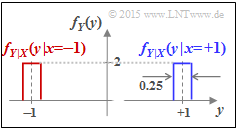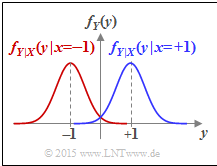# Difference between revisions of "Aufgaben:Exercise 4.9Z: Is Channel Capacity C ≡ 1 possible with BPSK?"Two different PDFs  $f_N(n)$  for the impairments  (e.g. noise)

We assume here a binary bipolar source signal   ⇒   $x \in X = \{+1, -1\}$.

Thus,  the probability density function  $\rm (PDF)$  of the source is:

$$f_X(x) = {1}/{2} \cdot \delta (x-1) + {1}/{2} \cdot \delta (x+1)\hspace{0.05cm}.$$

The mutual information between the source  $X$  and the sink  $Y$  can be calculated according to the equation

$$I(X;Y) = h(Y) - h(N)$$

where holds:

• $h(Y)$  denotes the  differential sink entropy
$$h(Y) = \hspace{0.1cm} - \hspace{-0.45cm} \int\limits_{{\rm supp}(f_Y)} \hspace{-0.35cm} f_Y(y) \cdot {\rm log}_2 \hspace{0.1cm} \big[ f_Y(y) \big] \hspace{0.1cm}{\rm d}y \hspace{0.05cm},$$
$${\rm with}\hspace{0.5cm} f_Y(y) = {1}/{2} \cdot \big[ f_{Y\hspace{0.05cm}|\hspace{0.05cm}{X}}(y\hspace{0.05cm}|\hspace{0.05cm}{X}=-1) + f_{Y\hspace{0.05cm}|\hspace{0.05cm}{X}}(y\hspace{0.05cm}|\hspace{0.05cm}{X}=+1) \big ]\hspace{0.05cm}.$$
• $h(N)$  gives the  differential noise entropy  computable from the PDF  $f_N(n)$  alone:
$$h(N) = \hspace{0.1cm} - \hspace{-0.45cm} \int\limits_{{\rm supp}(f_N)} \hspace{-0.35cm} f_N(n) \cdot {\rm log}_2 \hspace{0.1cm} \big[ f_N(n) \big] \hspace{0.1cm}{\rm d}n \hspace{0.05cm}.$$

Assuming a Gaussian distribution  $f_N(n)$  for the noise  $N$  according to the upper sketch,  we obtain the channel capacity  $C_\text{BPSK} = I(X;Y)$,  which is shown in the  theory section  depending on  $10 \cdot \lg (E_{\rm B}/{N_0})$ .

The question to be answered is whether there is a finite  $E_{\rm B}/{N_0}$  value for which  $C_\text{BPSK}(E_{\rm B}/{N_0}) ≡ 1 \ \rm bit/channel\:use$  is possible   ⇒   subtask  (5).

In subtasks  (1)  to  (4),  preliminary work is done to answer this question.  The uniformly distributed noise PDF  $f_N(n)$  is always assumed (see sketch below):

$$f_N(n) = \left\{ \begin{array}{c} 1/(2A) \\ 0 \\ \end{array} \right. \begin{array}{*{20}c} {\rm{f\ddot{u}r}} \hspace{0.3cm} |\hspace{0.05cm}n\hspace{0.05cm}| < A, \\ {\rm{f\ddot{u}r}} \hspace{0.3cm} |\hspace{0.05cm}n\hspace{0.05cm}| > A. \\ \end{array}$$

Hints:

### Questions

1

What is the differential entropy with the uniform PDF  $f_N(n)$  and  $\underline{A = 1/8}$?

 $h(N) \ = \$ $\ \rm bit/symbol$

2

What is the differential sink entropy with the uniform PDF  $f_N(n)$  and  $\underline{A = 1/8}$?

 $h(Y) \ = \$ $\ \rm bit/symbol$

3

What is the magnitude of the mutual information between the source and sink?  Assume further a uniformly distributed impairments with  $\underline{A = 1/8}$ .

 $I(X;Y) \ = \$ $\ \rm bit/symbol$

4

Under what conditions does the result of subtask  (3)  not change?

 For any  $A ≤ 1$  for the given uniform distribution. For any other PDF  $f_N(n)$,  limited to the range  $|\hspace{0.05cm}n\hspace{0.05cm}| < 1$ . If  $f_{Y\hspace{0.05cm}|\hspace{0.05cm}{X}}(y\hspace{0.08cm}|\hspace{0.05cm}{X}=-1)$  and  $f_{Y\hspace{0.05cm}|\hspace{0.05cm}{X}}(y\hspace{0.08cm}|\hspace{0.05cm}{X}=+1)$  do not overlap.

5

Now answer the crucial question, assuming,  that Gaussian noise is the only impairment and the quotient  $E_{\rm B}/{N_0}$  is finite.

 $C_\text{BPSK}(E_{\rm B}/{N_0}) ≡ 1 \ \rm bit/channel\:use$  is possible with a Gaussian PDF. For Gaussian noise with finite  $E_{\rm B}/{N_0}$ ,   $C_\text{BPSK}(E_{\rm B}/{N_0}) < 1 \ \rm bit/channel\:use$ is always valid.

### Solution

#### Solution

(1)  The differential entropy of a uniform distribution of absolute width  $2A$  is equal to

$$h(N) = {\rm log}_2 \hspace{0.1cm} (2A) \hspace{0.3cm}\Rightarrow \hspace{0.3cm} A=1/8\hspace{-0.05cm}: \hspace{0.15cm}h(N) = {\rm log}_2 \hspace{0.1cm} (1/4) \hspace{0.15cm}\underline{= -2\,{\rm bit/symbol}}\hspace{0.05cm}.$$PDF of the output variable  $Y$
with uniformly distributed noise  $N$

(2)  The probability density function at the output is obtained according to the equation:

$$f_Y(y) = {1}/{2} \cdot \big [ f_{Y\hspace{0.05cm}|\hspace{0.05cm}{X}}(y\hspace{0.05cm}|\hspace{0.05cm}x=-1) + f_{Y\hspace{0.05cm}|\hspace{0.05cm}{X}}(y\hspace{0.05cm}|\hspace{0.05cm}x=+1) \big ]\hspace{0.05cm}.$$

The graph shows the result for our example  $(A = 1/8)$:

• Drawn in red is the first term  ${1}/{2} \cdot f_{Y\hspace{0.05cm}|\hspace{0.05cm}{X}}(y\hspace{0.05cm}|\hspace{0.05cm}-1)$,  where the rectangle  $f_N(n)$  is shifted to the center position  $y = -1$  and is multiplied by  $1/2$ .  The result is a rectangle of width  $2A = 1/4$  and height  $1/(4A) = 2$.
• Shown in blue is the second term  ${1}/{2} \cdot f_{Y\hspace{0.05cm}|\hspace{0.05cm}{X}}(y\hspace{0.05cm}|\hspace{0.05cm}+1)$  centered at  $y = +1$.
• Leaving the colors out of account,  the total PDF  $f_Y(y)$ is obtained.
• The differential entropy is not changed by moving non-overlapping PDF sections.
• Thus, for the differential sink entropy we are looking for,  we get:
$$h(Y) = {\rm log}_2 \hspace{0.1cm} (4A) \hspace{0.3cm}\Rightarrow \hspace{0.3cm} A=1/8\hspace{-0.05cm}: \hspace{0.15cm}h(Y) = {\rm log}_2 \hspace{0.1cm} (1/2) \hspace{0.15cm}\underline{= -1\,{\rm bit/symbol}}\hspace{0.05cm}.$$

(3)  Thus, for the mutual information between source and sink, we obtain:

$$I(X; Y) = h(Y) \hspace{-0.05cm}-\hspace{-0.05cm} h(N) = (-1\,{\rm bit/symbol})\hspace{-0.05cm} -\hspace{-0.05cm}(-2\,{\rm bit/symbol}) \hspace{0.15cm}\underline{= +1\,{\rm bit/symbol}}\hspace{0.05cm}.$$

(4)  All the proposed solutions  are true:

• For each  $A ≤ 1$  holds
$$h(Y) = {\rm log}_2 \hspace{0.1cm} (4A) = {\rm log}_2 \hspace{0.1cm} (2A) + {\rm log}_2 \hspace{0.1cm} (2)\hspace{0.05cm}, \hspace{0.5cm} h(N) = {\rm log}_2 \hspace{0.1cm} (2A)$$
$$\Rightarrow \hspace{0.3cm} I(X; Y) = h(Y) \hspace{-0.05cm}- \hspace{-0.05cm}h(N) = {\rm log}_2 \hspace{0.1cm} (2) \hspace{0.15cm}\underline{= +1\,{\rm bit/symbol}}\hspace{0.05cm}.$$PDF of the output quantity  $Y$
with Gaussian noise  $N$
• This principle does not change even if the PDF  $f_N(n)$  is different,  as long as the noise is limited to the range  $|\hspace{0.05cm}n\hspace{0.05cm}| ≤ 1$ .
• However,  if the two conditional probability density functions overlap,  the result is a smaller value for  $h(Y)$  than calculated above and thus smaller mutual information.

(5)  Correct is the  proposed solution 2:

• The Gaussian function decays very fast,  but it never becomes exactly equal to zero.
• There is always an overlap of the conditional density functions  $f_{Y\hspace{0.05cm}|\hspace{0.05cm}{X}}(y\hspace{0.08cm}|\hspace{0.05cm}x=-1)$  and  $f_{Y\hspace{0.05cm}|\hspace{0.05cm}{X}}(y\hspace{0.08cm}|\hspace{0.05cm}x=+1)$.
• According to subtask  (4) ,  $C_\text{BPSK}(E_{\rm B}/{N_0}) ≡ 1 \ \rm bit/channel\:use$  is therefore not possible.